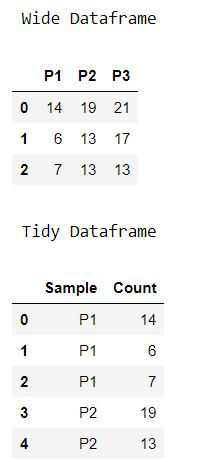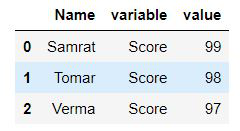Open in App
Not now

# Reshape Wide DataFrame to Tidy with identifiers using Pandas Melt

• Last Updated : 02 Feb, 2021

Sometimes we need to reshape the Pandas data frame to perform analysis in a better way. Reshaping plays a crucial role in data analysis. Pandas provide functions like melt and unmelt for reshaping. In this article, we will see what is Pandas Melt and how to use it to reshape wide to Tidy with identifiers.

Pandas Melt(): Pandas.melt() unpivots a DataFrame from wide format to long format. Pandas melt() function is utilized to change the DataFrame design from wide to long. It is utilized to make a particular configuration of the DataFrame object where at least one segments fill in as identifiers. All the rest of the sections are treated as qualities and unpivoted to the line pivot and just two segments, variable and worth.

Syntax: Pandas.melt(column_level=None, variable_name=None, Value_name=’value’, value_vars=None, id_vars=None, frame)

Parameters:

• frame : DataFrame
• id_vars[tuple, list, or ndarray, optional]: Column(s) to use as identifier variables.
• value_vars[tuple, list, or ndarray, optional]: Column(s) to unpivot. If not specified, uses all columns that are not set as id_vars.
• var_name[scalar]: Name to use for the ‘variable’ column. If None it uses frame.columns.name or ‘variable’.
• value_name[scalar, default ‘value’]: Name to use for the ‘value’ column.
• col_level[int or string, optional]: If columns are a MultiIndex then use this level to melt.

Example 1:

## Python3

 `# Load the libraries ` `import` `numpy as np ` `import` `pandas as pd ` `from` `scipy.stats ``import` `poisson ` ` `  `# We will use scipy.stats to create ` `# random numbers from Poisson distribution. ` `np.random.seed(seed ``=` `128``) ` `p1 ``=` `poisson.rvs(mu ``=` `10``, size ``=` `3``) ` `p2 ``=` `poisson.rvs(mu ``=` `15``, size ``=` `3``) ` `p3 ``=` `poisson.rvs(mu ``=` `20``, size ``=` `3``) ` ` `  `# Declaring the dataframe ` `data ``=` `pd.DataFrame({``"P1"``:p1, ` `                 ``"P2"``:p2, ` `                 ``"P3"``:p3}) ` ` `  `# Dataframe ` `print``(``" Wide Dataframe"``) ` `display(data) ` ` `  `data.melt() ` ` `  `# Change the names of the columns ` `data.melt(var_name ``=` `[``"Sample"``]).head() ` ` `  `# Specify a name for the values ` `print``(``"\n Tidy Dataframe"``) ` `data.melt(var_name ``=` `"Sample"``, ` `          ``value_name ``=` `"Count"``).head() `

Output:Explanation: In this example, we create three datasets using Poisson distribution and create a data frame using pandas. Then using the melt() function we reshape the data in long-form in two columns and rename the two columns. The first column is called “variable” by default and it contains the column/variable names. And the second column is named “value” and it contains the data from the wide form data frame.

Example 2:

## Python3

 `import` `pandas as pd ` ` `  ` `  `data ``=` `pd.DataFrame({``'Name'``: {``0``: ``'Samrat'``, ``1``: ``'Tomar'``, ``2``: ``'Verma'``}, ` `                     ``'Score'``: {``0``: ``'99'``, ``1``: ``'98'``, ``2``: ``'97'``}, ` `                     ``'Age'``: {``0``: ``22``, ``1``: ``31``, ``2``: ``33``}}) ` ` `  `pd.melt(data, id_vars``=``[``'Name'``], value_vars``=``[``'Score'``]) ` ` `  `display(pd.melt(data, id_vars``=``[``'Name'``], value_vars``=``[``'Score'``]))`

Output:Explanation: In this example, we create a data frame using pandas. Then using the melt() function we reshape the data in long-form in three columns and specify the Name as the id and variable as Score the person and the value as their scores. Apart from the “id” column, The first column is called “variable” by default and it contains the column/variable names. And the second column is named “value” and it contains the data from the wide form data frame.

My Personal Notes arrow_drop_up
Related Articles